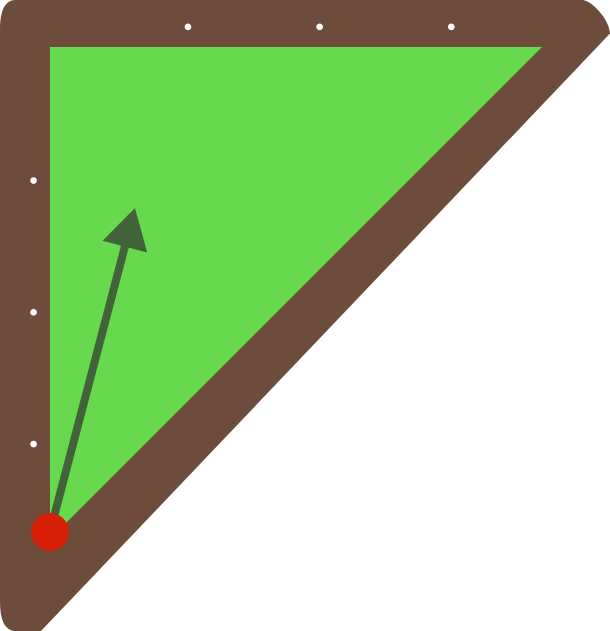Back to Open Problems Group feed

# Triangular Pool Bounce

Geometry Level 4

Suppose you have a triangular pool table with a ball starting at the marked vertex of the billiard table shown (shaped like an isosceles right triangle). Is it possible to hit a ball that reflects back to where it started (given any number of reflections)?Assume geometric purity: the "ball" has no mass and the "ball" and "hole" are considered single points. Also, if the ball hits a vertex point as opposed to a side, then the reflections are absorbed. (This means hitting straight up towards the right angle won't work.)

This problem is part of the Brilliant.org Open Problems Group. The end goal for each open problem is to find a solution, and maybe publish it if it's a nice enough result! You can read about the unsolved billiards problem that this one is related to here.

×

Problem Loading...

Note Loading...

Set Loading...